# Linear Wave Shaping

## Double Differentiation – Derivation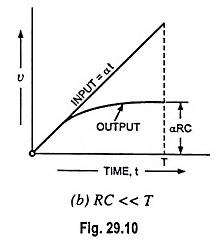Double Differentiation – Derivation: Two R-C coupling networks in cascade separated by an ampli­fier A is shown in Fig. 29.36 (a). The amplifier A is to operate as a linear amplifier and the output impedance must be small with respect to the impedance of R2 and C2 so that this combination does not load the amplifier. …

## RC Integrator Circuit Diagram and its Application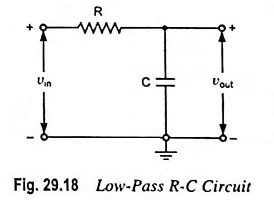RC Integrator Circuit Diagram and its Application: A circuit that gives an output voltage directly proportional to the integral of its input is known as integrating circuit. Figure 29.18 shows a typical RC Integrator Circuit diagram. The output voltage across capacitor C will be the integral of the input volt­age. It is important to note …

## Low Pass RC Circuit Diagram, Derivation and Application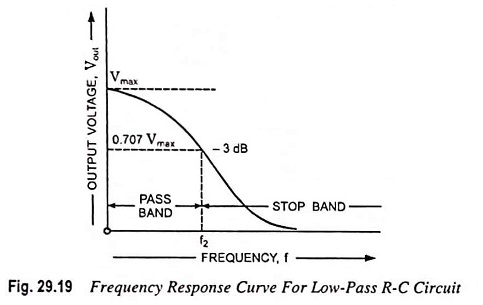Low Pass RC Circuit Diagram, Derivation and Application: The Low Pass RC Circuit is shown in Fig. 29.18. In a Low Pass RC Circuit, the output voltage vout is taken across the capacitor. Resist­ance offers fixed opposition. Since the reactance offered by the capacitor C falls with the increase in frequency, low frequency signal develops across …

## RC High Pass Circuit as Differentiator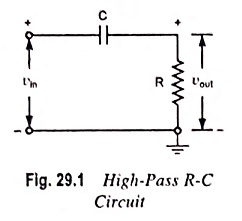RC High Pass Circuit as Differentiator: RC High Pass Circuit as Differentiator – A circuit that gives an output voltage proportional to the deriva­tive of its input, is known as a Differentiator circuit. Figure 29.1 shows a typical RC High Pass Circuit as Differentiator. The out­put voltage across R will be the derivative of the input …

## High Pass RC Circuit Diagram, Derivation and Application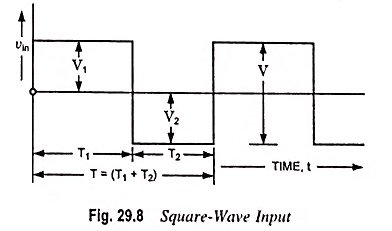High Pass RC Circuit Diagram, Derivation and Application: The High Pass RC Circuit is shown in Fig. 29.1. The reactance of the capacitor is given as i.e., Reactance XC of the circuit capacitor decreases with the increase in frequency. Thus at low frequencies the capacitor C offers considerable reactance and so blocks them, but at …

Scroll to Top